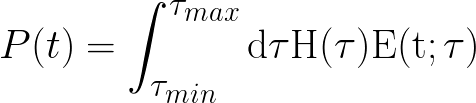# RooFFTConvPdf: p.d.f normalization integral is zero or

Dear all,
I am trying to convolve a RooExponential with a RooHistPdf; the time constant tau of the RooExponential is
distributed according to a RooHistPdf.
I want then to generate some data from the projected convolved distribution.
I have followed the useful example rf211_paramconv.C.

When I try to run my code (attached here together with the needed data for the tau distribution),
I get the following error:

```[#0] ERROR:Eval -- RooAbsReal::logEvalError(tauHPDF_fft) evaluation error, origin : RooHistPdf::tauHPDF_fft[ pdfObs=(tau_shifted_FFTBuffer2) ] message : p.d.f normalization integral is zero or negative server values: pdfObs=(tau_shifted_FFTBuffer2 = -1.485)```

at this line:

```RooDataHist* d = projtrapConv->generateBinned(t,1000) ; ```

the error gets repeated over and over.

Later on other errors appear, like:

```[#0] ERROR:Eval -- RooAbsReal::logEvalError(trapConv_Int[tau]_Norm[t,tau]) evaluation error, origin : RooRealIntegral::trapConv_Int[tau]_Norm[t,tau][ Int trapConv_Norm(t,tau) d[Ana](tau) ] message : function value is NAN server values: !sumList=(), !intList=(), !anaList=(tau = -2.51149), !jacList=(), !facList=(), !func=trapConv=0, !sumCat=()```

Am I missing something?
I am using the following root version:

``````   ------------------------------------------------------------
| Welcome to ROOT 6.02/04                http://root.cern.ch |
|                               (c) 1995-2014, The ROOT Team |
| Built for macosx64                                         |
| From tag v6-02-04, 17 January 2015                         |
| Try '.help', '.demo', '.license', '.credits', '.quit'/'.q' |
------------------------------------------------------------``````

Any help will be greatly appreciated!

Many thanks in advance and best regards,
Marco Bomben
tests_140224-fei4-250um-fl3.8e15-extract_traps-V400-taue-in-ns.root (3.81 KB)
convolution_hist.C (3.43 KB)

I will tell our expert to look at it.
I am myself not an expert but looking to your output I see that you have a range with negative numbers:

``````mean = -2.51149
xmin = -3
xmax = -1.5``````

and then it seems that you are doing some kind of log evaluation:

``[#0] ERROR:Eval -- RooAbsReal::logEvalError(tauHPDF_fft) evaluation error, ``

It is well known than negative numbers and log are not good friends… could it be the problem ? (just a suggestion … I cannot help you further).

Dear Olivier,
the time constant tau of the exponential is indeed negative definite as I need a monotonically decreasing function. If I understand correctly this is the way it works in RooFit if you need an exponential decay function.

Thanks,
Marco

Ok, Lorenzo will reply when back next week.

Hi,

Your model seems to be ill formed to me, for this you are getting NaN. Can you please write the mathematical expression of your model , i.w. what is the equation of your pdf ?

Thank you

Lorenzo

Dear Lorenzo,

in the attached picture you can see which pdf I am trying to model: it is the convolution of a RooExponential E(t;tau) with a RooHistPdf H(tau) which is based on an histogram; the histogram contains the values for tau.

Best regards,
Marco BombenHi,

What you wrote is not exactly a convolution. A convolution is defined as

en.wikipedia.org/wiki/Convolution

I don’t think you can use the RooFFTConvPdf in your case for this integral. You should probably use the RooProdPdf and then compute the projection in tau

Best Regards

Lorenzo

Many thanks for the explanation. I have used RooProdPdf and it works as expected.
You can consider this issue closed.

Many thanks and best regards,
Marco Bomben